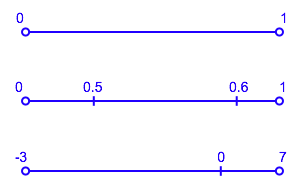# Coordinate Systems and Metrics

In the previous Poincaré Project post about coordinate systems, I introduced two charts that covered the circle and introduced a coordinate system ranging from 0 to 1 on each of them.

At the time I said:

I've arbitrarily ranged the two coordinate systems from 0 to 1 but any continuous parameterisation of the coordinate curve would work.

Here are three examples of different coordinate systems on the blue chart:The point to show here is that not only is the choice of the end points arbitrary but that the coordinate can change at a different rate depending on where you are. In the second example, the distance between 0.5 and 0.6 is great than that between 0.4 and 0.5 or 0.5 and 0.6.

Notice that we haven't yet introduced any structure to the manifold itself that allows as to talk about distance without (as we've done on this web page) actually identifying the points in our manifold with points in a higher-dimensional euclidian space (in this case the R^2 of the screen).

This notion of the "rate of change" of the coordinate function lets us do so intrinsically, however. Say the distance between the point 0.5 and 0.6 is 5 in some units. Then the ratio of distance to change of coordinate is 5 / (0.6 - 0.5) = 50. Do this again from 0.5 to 0.55, or from 0.5 to 0.51 or from 0.5 to 0.5001. In other words, take the limit to a change in coordinate of 0.

This tells us how much distance is travelled as a proportion of coordinate change at the point 0.5. This "distance travelled as proportion of coordinate change" is called a metric for that coordinate system. Note that it's a function of the point you're at.

If you want to calculate the distance between arbitrary points with coordinates a and b, you take the integral of this metric function between a and b.

This new structure of a manifold with a metric defined on each chart is core not only to the proof of the Poincaré Conjecture but also to Einstein's General Theory of Relativity. In fact, by the time we've covered what we need for Poincaré, we will have covered more than enough mathematics to understand GR too.

One final thought: notice that, in our example, the metric isn't really telling you much about the manifold itself at all. The metric is merely expressing the relationship between the coordinate system and the notion of distance. We'll see shortly, however, that in dimensions higher that one, it goes beyond just telling you something about the coordinate system and allows you to describe the curvature intrinsic to the manifold.

UPDATE: next post

The original post was in the category: poincare_project but I'm still in the process of migrating categories over.